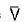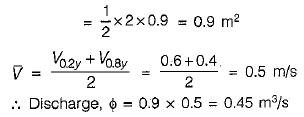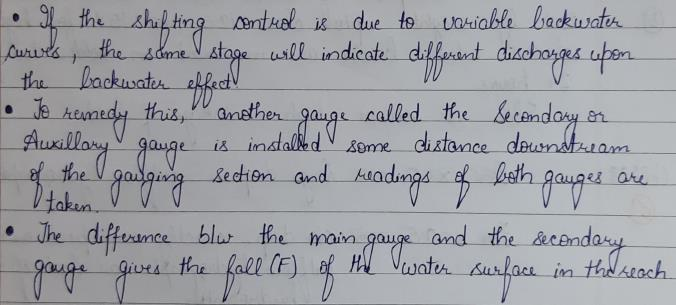Courses

# Test: Streamflow Measurement - 1

## 10 Questions MCQ Test GATE Civil Engineering (CE) 2022 Mock Test Series | Test: Streamflow Measurement - 1

Description
This mock test of Test: Streamflow Measurement - 1 for Civil Engineering (CE) helps you for every Civil Engineering (CE) entrance exam. This contains 10 Multiple Choice Questions for Civil Engineering (CE) Test: Streamflow Measurement - 1 (mcq) to study with solutions a complete question bank. The solved questions answers in this Test: Streamflow Measurement - 1 quiz give you a good mix of easy questions and tough questions. Civil Engineering (CE) students definitely take this Test: Streamflow Measurement - 1 exercise for a better result in the exam. You can find other Test: Streamflow Measurement - 1 extra questions, long questions & short questions for Civil Engineering (CE) on EduRev as well by searching above.
QUESTION: 1

Solution:
QUESTION: 2

### The following is not a direct stream flow determination technique:

Solution:

Indirect methods are those methods which make use of relationship between the flow discharger and the depths at specified locations. Two types of these indirect method are flow measuring structures and slope area methods.

QUESTION: 3

### A stilling well is required when the stage measurement is made by employing a

Solution:

The float-operated stage recorder is the most common type of automatic stage recorder in use. In this a float operating in a silting well is balanced by means of a counterweight over the pulley of a recorder, To protect the float from debris and to reduce the water surface wave effects on the recording, silting wells are provided in all float- type stage recorder installations.

QUESTION: 4

In a triangular channel the top width and depth of flow were 2.0 m and 0.9 m respectively. Velocity measurements on the centre line at 18 cm and 72 cm below water surface indicated velocities of 0.60 m/s and 0.40 m/s respectively. The discharge in the channel (in m3/s) is

Solution:

Discharge in the channel
=x area of channel Area of channel

Area of channelQUESTION: 5

In the moving-boat method of stream-flow measurement, the essential measurements are

Solution:

The moving-boat technique measures the velocity over the width of a segment by suspending the current meter at a constant depth during the transverse of the boat across the stream.
The measured velocity and the additional information of the depth gives the discharge.
Thus, velocity of the river is determined by whether the current meter measures along or opposite of the stream and the velocity it measures.
The depth multiplied with velocity gives volume per unit width of water flown. The volume divided with time gives discharge flown.

QUESTION: 6

Which of the following instruments in not connected with stream flow measurement?

Solution:
QUESTION: 7

The surface velocity at any vertical section of a stream is

Solution:
QUESTION: 8

If a gauging section is having shifting control due to backwater effects, then

Solution:QUESTION: 9

The stage discharge relation in a river during the passage of a flood wave is measured. If QR = discharge at a stage when the water surface was rising and Q= discharge at the same stage when the water surface was falling, then

Solution:
QUESTION: 10

The dilution method of stream gauging is ideally suited for measuring discharges in

Solution:

The dilution method has the major disadvantage that the discharge is estimated directly in an absolute way. It is a particularly attractive method for small turbulent streams, such as these in mountainous areas.